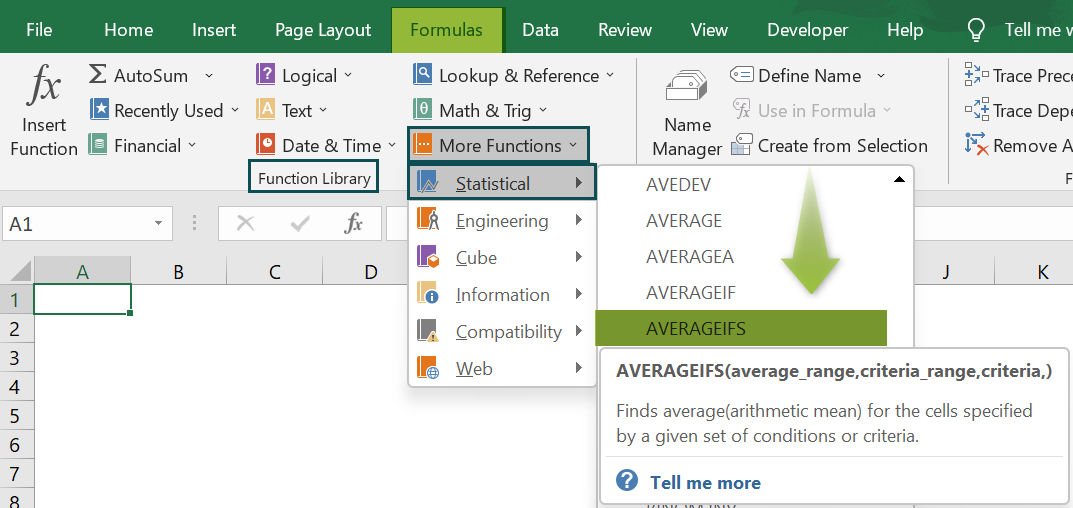# AVERAGEIFS Excel FunctionArticle byExcelMojo Team## What Is AVERAGEIFS Function In Excel?

The AVERAGEIFS Function in Excel calculates the average/mean of the numeric values that match multiple criteria given for a selected dataset. The logical operators (>,<,<>,=) and wildcards (*,?) can be used by the AVERAGEIFS function just like the SUMIFS and COUNTIFS functions. The AVERAGEIFS Excel function is an inbuilt Statistical function, so we can insert the formula from the “Function Library” or enter it directly in the worksheet.

For example, the following image shows values in columns A and B. We will calculate the average of the numbers based on set criteria using the AVERAGEIFS Excel function.

Select cell B8, enter the formula =AVERAGEIFS(B2:B7,A2:A7,”A”), and press “Enter”. The result is ‘59.333’, as shown below.

###### Key Takeaways
• The AVERAGEIFS Excel function helps users provide multiple criteria for a given dataset, finds the cells that fulfill all the criteria, and calculates the average of the respective cell values.
• The function treats the cell as a ‘0’ value if any cell in the criteria range is empty.
• The AVERAGEIFS evaluates the cell range that contains TRUE as 1, and FALSE as 0.
• We can use the conditional operators (>,<,<>,=) or special characters (?,*) to match partial conditions or to include certain conditions in the formula.

### AVERAGEIFS() Excel Formula

The syntax of the AVERAGEIFS Excel formula is,

The arguments of the AVERAGEIFS Excel formula are,

• average_range: It is a mandatory argument. It is the cell rage to find the average.
• criteria_range1: It is a mandatory argument. It is a cell range to apply the criteria.
• criteria1: It is a mandatory argument. It is the first criteria for the first cell range from the given dataset, followed by [criteria_range2,criteria2], [criteria_range3,criteria3], and so on

### How To Use AVERAGEIFS Excel Function?

We can use the AVERAGEIFS function in Excel in two ways, namely,

1. Access from the Excel ribbon.
2. Enter in the worksheet manually.

#### Method #1 – Access from the Excel ribbon

Choose an empty cell for the output → select the “Formulas” tab → go to the “Function Library” group → click the “More Functions” option drop-down → click the “Statistical” option right arrow → select the “AVERAGEIFS” function, as shown below.

The “Function Arguments” window appears. Enter the arguments in the “Average_range”, “Criteria_range1”, and “criteria” fields → click “OK”, as shown below.

[Note: To view the “Criteria1” field, click the “Criteria_range1” field.]

#### Method #2 – Enter in the worksheet manually

1. Select an empty cell for the output.
2. Type =AVERAGEIFS( in the cell. [Alternatively, type =A and double-click the AVERAGEIFS function from the Excel suggestions.]
3. Enter the arguments as cell values or cell references in Excel.
4. Close the brackets of the formula.
5. Press the “Enter” key.

Let us take a basic example to understand this function.

Here, we will calculate the average of the numbers based on set criteria using AVERAGEIFS Excel function for the zonal-wise employee sales.

In the table, the data is,

• Column A contains the Zone.
• Column B contains the Employee.
• Column C contains the Sales.

The steps to calculate the average using AVERAGEIFS Excel function are,

1. Select cell C14, and enter the formula =AVERAGEIFS(C2:C13, i.e., the average_range value.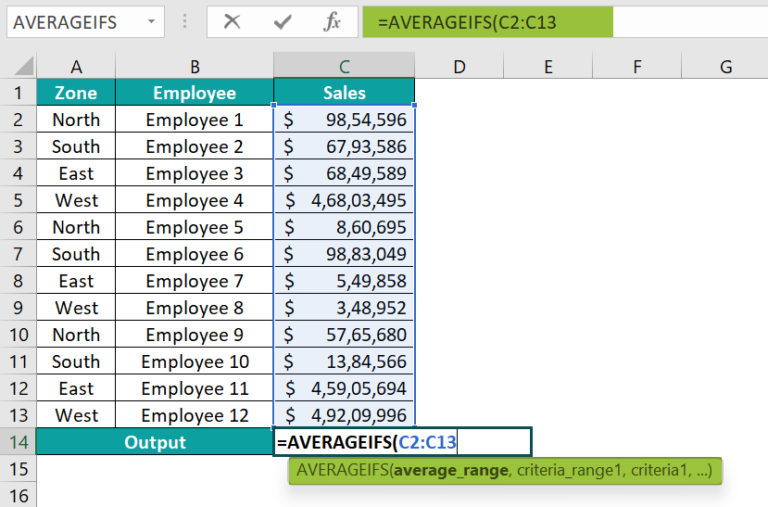2. In continuation with the formula, enter the value of ‘criteria_range1’ as A2:A13. So, the formula is =AVERAGEIFS(C2:C13,A2:A13,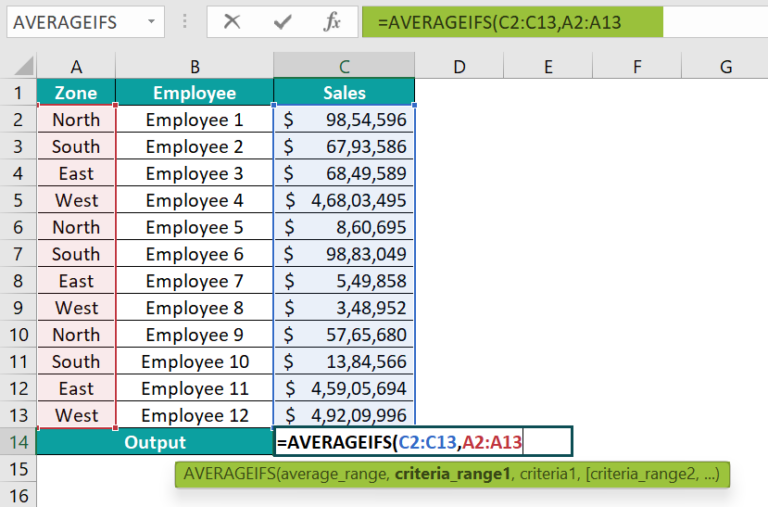3. In continuation with the formula, enter the value of ‘criteria1’ as North, and close the brackets. The complete formula is =AVERAGEIFS(C2:C13,A2:A13,”North”), as shown below.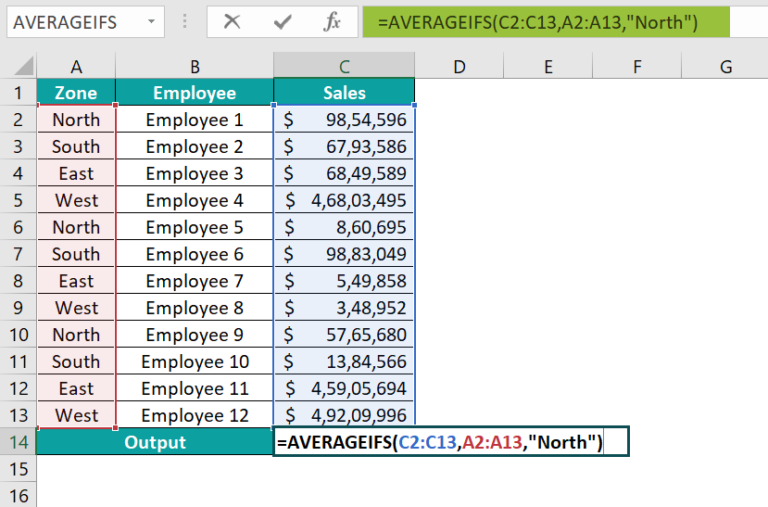4. Press the “Enter” key. The result is “\$54,93,657”, as shown below.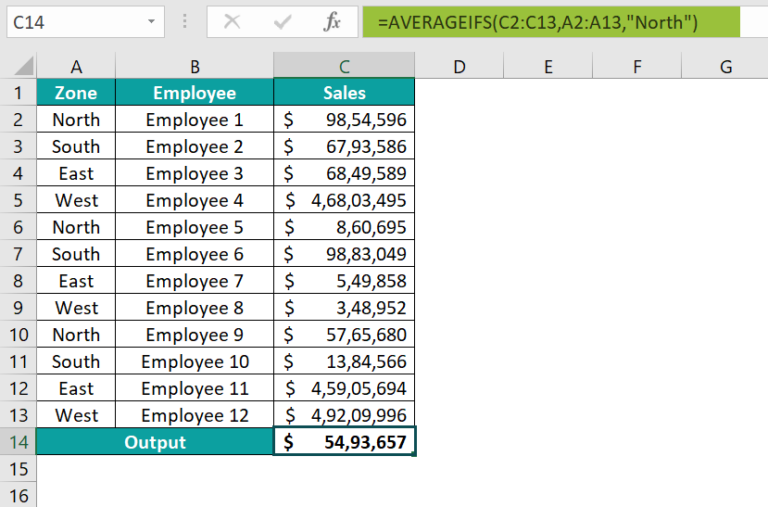### Examples

We will understand some advanced scenarios using AVERAGEIFS Excel function examples.

#### Example #1

We will calculate the average of the numbers based on set criteria using the AVERAGEIFS function for the product quantity.

In the table, the data is,

• Column A contains the Product.
• Column B contains the Quantity.

The procedure to calculate the average using the AVERAGEIFS Excel function is,

Select cell B7, enter the formula =AVERAGEIFS(B2:B6,A2:A6,”Bakery”), and press the “Enter” key.

The result is “6566”, as shown above.

#### Example #2

We will calculate the average of the numbers based on set criteria using AVERAGEIFS Excel function for the student marks.

In the table, the data is,

• Column A contains the Class.
• Column B contains the Marks.

The procedure to calculate the average using the AVERAGEIFS Excel function is,

Select cell B7, enter the formula =AVERAGEIFS(B2:B6,A2:A6,”Class I”), and press the “Enter” key.

The result is “7.667”, as shown above.

#### Example #3

We will calculate the average of the numbers based on multiple criteria using the AVERAGEIFS formula for the medals won by countries in the tennis tournament, and

In the table, the data is,

• Column A contains the Countries.
• Column B contains the Result.
• Column C contains the Medals.

The steps to calculate the average with multiple criteria using the AVERAGEIFS Function are,

• Step 1: Select cell C7, enter the formula =AVERAGEIFS(C2:C6,B2:B6,”Won”

[Note: The first criteria, i.e., criteria1, is to check for “Won” in the criteria_range1, B2:B6.]

• Step 2: In continuation with the formula, select the criteria_range2, A2:A6, and the second criteria, i.e., criteria2 as *a*, which will check for the country names having the letter ‘a, and close the brackets.

Therefore, the complete formula is =AVERAGEIFS(C2:C6,B2:B6,”=Won”,A2:A6,”*a*”)

• Step 3: Press the “Enter” key.

The result is “6.333333333”, as shown above.

[Output Observation: The formula first checks whether cell range C2:C6 satisfies both conditions.

• Condition 1: The cells C2, C4, and C5, have the text string “Won” in their cells B2, B4, and B5, respectively.
• Condition 2: Their respective countries in cells A2, A4, and A5, i.e., Brazil, Italy, and India, have the letter ‘a’ in their names.

Therefore, the cell values that satisfy both conditions are C2, C4, and C5. Now, the formula calculates the average,i.e., (C2+C4+C5)/3, which is (5+4+10)/3 or 19/3=6.33333.]

### Important Things To Note

• The #DIV/0! error occurs when:
• The “average_range” argument is left blank or is a text value.
• None of the criteria are met.
• Each “criteria_range” provided in the formula must be the same size, else we will get the #VALUE!” error.
• We will get a “#NAME?” error if the function’s name is incorrectly entered in the formula or if we do not close the brackets of the formula.

1. What does the AVERAGEIFS function do in Excel?

The AVERAGEIFS function calculates the average of cells that fulfills all the given criteria or conditions.

The syntax of the AVERAGEIFS function is =AVERAGEIFS(average_range,criteria_range1,criteria1,[criteria_range2,criteria2]…)

2. How to use Excel AVERAGEIFS function?

The step-by-step procedure to calculate the average using the AVERAGEIFS Excel function is,

1. Select an empty cell for the output.
2. Type =AVERAGEIFS( in the cell. [Alternatively, type =A and double-click the AVERAGEIFS function from the Excel suggestions.]
3. Enter the arguments as cell values or cell references.
4. Close the brackets of the formula.
5. Press the “Enter” key.

For example, we will calculate the average of the numbers based on set criteria using the AVERAGEIFS formula for the people’s work done.
In the table, the data is,
Column A contains the Name.
Column B contains the Value.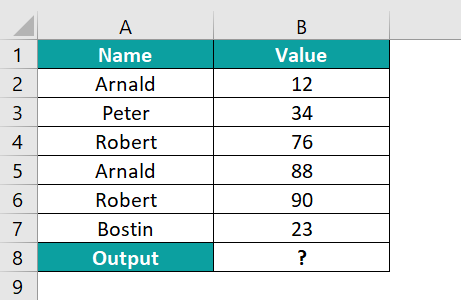The procedure to calculate the average using the AVERAGEIFS Excel function is,

Select cell B8, enter the formula =AVERAGEIFS(B2:B7,A2:A7,”Arnald”), and press the “Enter” key.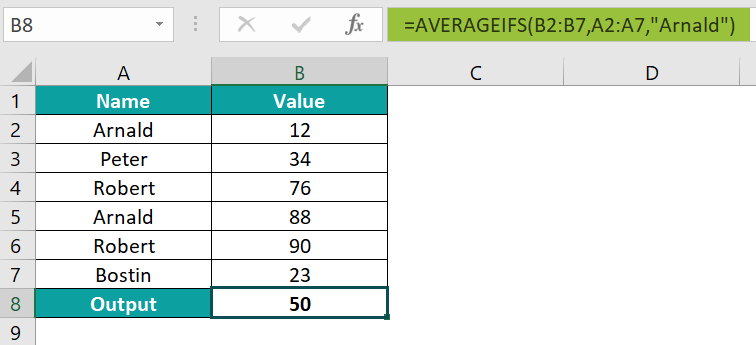The result is “50”, as shown above.

3. Where is the AVERAGEIFS function in Excel?

The AVERAGEIFS Excel function is found in the Formulas tab” as follows:

Choose an empty cell for the output → select the “Formulas” tab → go to the “Function Library” group → click the “More Functions” option drop-down → click the “Statistical” option right arrow → select the “AVERAGEIFS” function, as shown below.